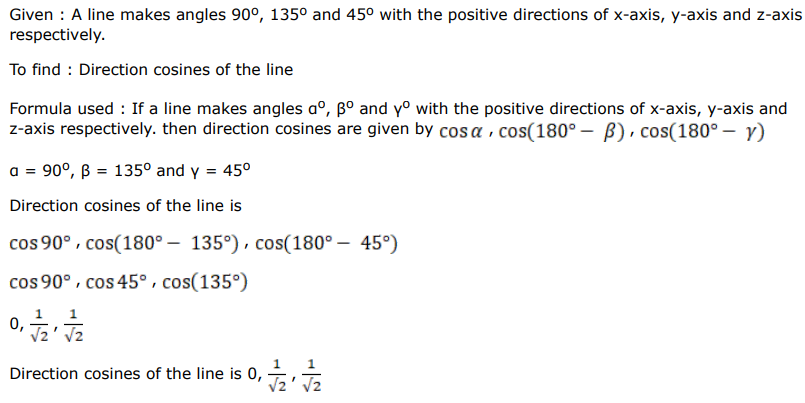# Solve this followingQuestion:

A line makes angles $90^{\circ}, 135^{\circ}$ and $45^{\circ}$ with the positive directions of $x$-axis, $y$-axis and $z$-axis respectively. what are the direction cosines of the line?

Solution: